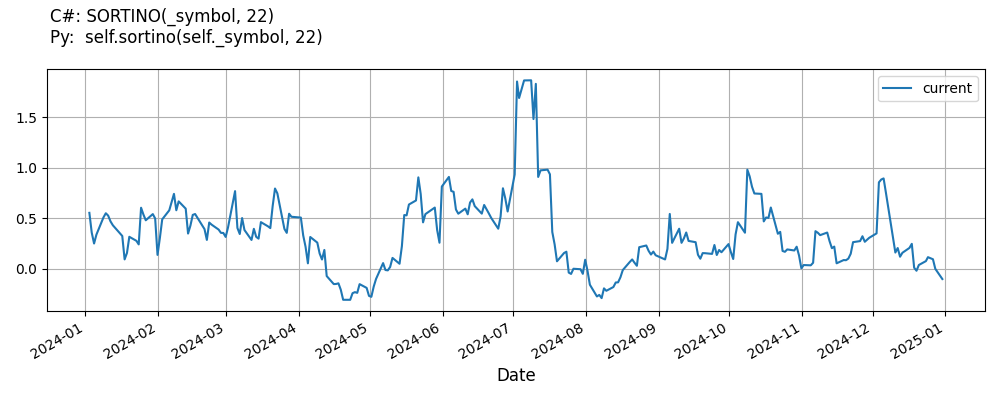# Supported Indicators

## Sortino Ratio

### Introduction

Calculation of the Sortino Ratio, a modification of the . Reference: https://www.cmegroup.com/education/files/rr-sortino-a-sharper-ratio.pdf Formula: S(x) = (R - T) / TDD Where: S(x) - Sortino ratio of x R - the average period return T - the target or required rate of return for the investment strategy under consideration. In Sortino’s early work, T was originally known as the minimum acceptable return, or MAR. In his more recent work, MAR is now referred to as the Desired Target Return. TDD - the target downside deviation.

To view the implementation of this indicator, see the LEAN GitHub repository.

### Using SORTINO Indicator

To create an automatic indicators for SortinoRatio, call the SORTINO helper method from the QCAlgorithm class. The SORTINO method creates a SortinoRatio object, hooks it up for automatic updates, and returns it so you can used it in your algorithm. In most cases, you should call the helper method in the Initialize method.

public class SortinoRatioAlgorithm : QCAlgorithm
{
private Symbol _symbol;
private SortinoRatio _sortino;

public override void Initialize()
{
_sortino = SORTINO(_symbol, 22);
}

public override void OnData(Slice data)
{
{
// The current value of _sortino is represented by itself (_sortino)
// or _sortino.Current.Value
Plot("SortinoRatio", "sortino", _sortino);

}
}
}
class SortinoRatioAlgorithm(QCAlgorithm):
def Initialize(self) -> None:
self.sortino = self.SORTINO(self.symbol, 22)

def OnData(self, slice: Slice) -> None:
# The current value of self.sortino is represented by self.sortino.Current.Value
self.Plot("SortinoRatio", "sortino", self.sortino.Current.Value)



The following reference table describes the SORTINO method:

### SORTINO()1/1

            SortinoRatio QuantConnect.Algorithm.QCAlgorithm.SORTINO (
Symbol                           symbol,
Int32                            sortinoPeriod,
*Double                          minimumAcceptableReturn,
*Nullable<Resolution>      resolution,
*Func<IBaseData, Decimal>  selector
)


Creates a new Sortino indicator.

If you don't provide a resolution, it defaults to the security resolution. If you provide a resolution, it must be greater than or equal to the resolution of the security. For instance, if you subscribe to hourly data for a security, you should update its indicator with data that spans 1 hour or longer.

You can manually create a SortinoRatio indicator, so it doesn't automatically update. Manual indicators let you update their values with any data you choose.

Updating your indicator manually enables you to control when the indicator is updated and what data you use to update it. To manually update the indicator, call the Update method with time/number pair, or an IndicatorDataPoint. The indicator will only be ready after you prime it with enough data.

public class SortinoRatioAlgorithm : QCAlgorithm
{
private Symbol _symbol;
private SortinoRatio _sortino;

public override void Initialize()
{
_sortino = new SortinoRatio(22);
}

public override void OnData(Slice data)
{
if (data.Bars.TryGeValue(_symbol, out var bar))
{
_sortino.Update(bar.EndTime, bar.Close);
}

{
// The current value of _sortino is represented by itself (_sortino)
// or _sortino.Current.Value
Plot("SortinoRatio", "sortino", _sortino);

}
}
}
class SortinoRatioAlgorithm(QCAlgorithm):
def Initialize(self) -> None:
self.sortino = SortinoRatio(22)

def OnData(self, slice: Slice) -> None:
bar = slice.Bars.get(self.symbol)
if bar:
self.sortino.Update(bar.EndTime, bar.Close)

# The current value of self.sortino is represented by self.sortino.Current.Value
self.Plot("SortinoRatio", "sortino", self.sortino.Current.Value)



To register a manual indicator for automatic updates with the security data, call the RegisterIndicator method.

public class SortinoRatioAlgorithm : QCAlgorithm
{
private Symbol _symbol;
private SortinoRatio _sortino;

public override void Initialize()
{
_sortino = new SortinoRatio(22);
RegisterIndicator(_symbol, _sortino, Resolution.Daily);
}

public override void OnData(Slice data)
{
{
// The current value of _sortino is represented by itself (_sortino)
// or _sortino.Current.Value
Plot("SortinoRatio", "sortino", _sortino);

}
}
}
class SortinoRatioAlgorithm(QCAlgorithm):
def Initialize(self) -> None:
self.sortino = SortinoRatio(22)
self.RegisterIndicator(self.symbol, self.sortino, Resolution.Daily)

def OnData(self, slice: Slice) -> None:
# The current value of self.sortino is represented by self.sortino.Current.Value
self.Plot("SortinoRatio", "sortino", self.sortino.Current.Value)



The following reference table describes the SortinoRatio constructor:

### SortinoRatio()1/2

            SortinoRatio QuantConnect.Indicators.SortinoRatio (
string   name,
int      period,
*double  minimumAcceptableReturn
)


Creates a new Sortino Ratio indicator using the specified periods.

### SortinoRatio()2/2

            SortinoRatio QuantConnect.Indicators.SortinoRatio (
int      period,
*double  minimumAcceptableReturn
)


Creates a new SortinoRatio indicator using the specified periods.

### Visualization

The following image shows plot values of selected properties of SortinoRatio using the plotly library.You can also see our Videos. You can also get in touch with us via Discord.